# Perimeter

Perimeter is the distance around a two-dimensional shape.

### Example: the perimeter of this rectangle is 7+3+7+3 = 20### Example: the perimeter of this regular pentagon is:

3+3+3+3+3 = 5×3 = 15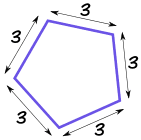The perimeter of a circle is called the circumference: Circumference = 2π × radius## Perimeter Formulas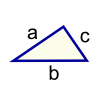Triangle Perimeter = a + b + c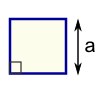Square Perimeter = 4 × a a = length of sideRectangle Perimeter = 2 × (a + b)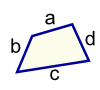Quadrilateral Perimeter = a + b + c + d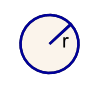Circle Circumference = 2πr r = radius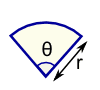Sector Perimeter = r(θ+2) r = radius θ = angle in radiansEllipse Perimeter = very hard!